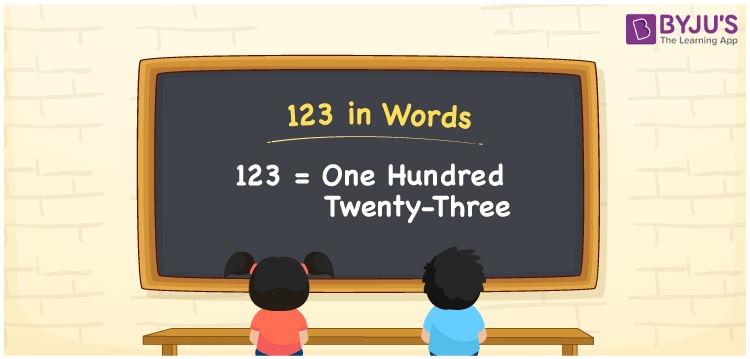# 123 in Words

123 in words is written as One hundred twenty-three. In both the International System of Numerals and the Indian System of Numerals, 123 is written as One hundred twenty-three. The number 123 is a Cardinal Number as it represents some quantity. For example, “we need 123 more plates”.

 123 in Words One hundred twenty-three One hundred twenty-three in Number 123

## 123 in English Words

We write 123 in English Words using the letters of the English alphabet. Therefore, we read 123 in English as “One hundred twenty-three.”## How to Write 123 in Words?

To write 123 in words, we shall use the place value chart. In the place value chart, write 1 in the hundreds, 2 in the tens, and 3 in the ones, respectively. Now let us make a place value chart to write the number 123 in words.

 Hundreds Tens Ones 1 2 3

Thus, we can write the expanded form as

1 × Hundred + 2 × Ten + 3 × One

= 1 × 100 + 2 × 10 + 3 × 1

= 100 + 20 + 3

= 123

= One hundred twenty-three.

123 is a natural number, the successor of 122 and the predecessor of 124.

123 in words – One hundred twenty-three

• Is 123 an odd number? – Yes
• Is 123 an even number? – No
• Is 123 a perfect square number? – No
• Is 123 a perfect cube number? – No
• Is 123 a prime number? – No
• Is 123 a composite number? – Yes

## Frequently Asked Questions on 123 in Words

### How to write 123 in words?

123 in words is written as Five Hundred.

### How to write 123 in words in the International and Indian System of Numerals?

In both the system of numerals, 123 is written as One hundred twenty-three.

### What are the factors of 123?

The factors of 123 are 1, 3, 41 and 123.